Скачать презентацию Chapter 10 Price Competition 1

• Количество слайдов: 34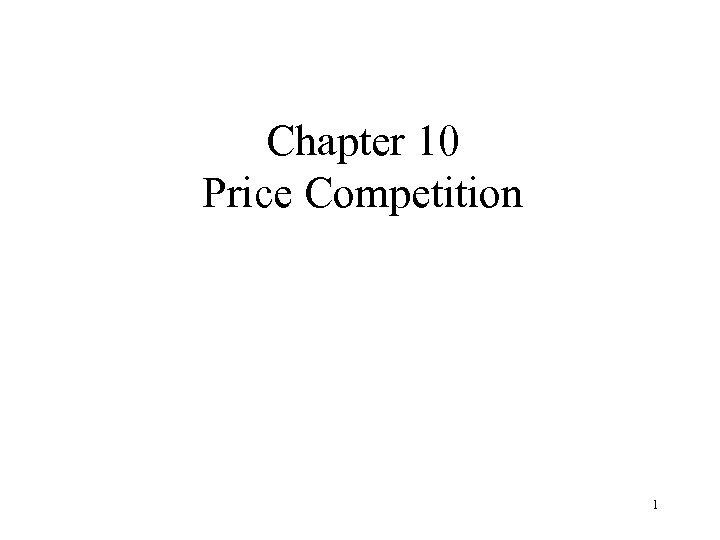Chapter 10 Price Competition 1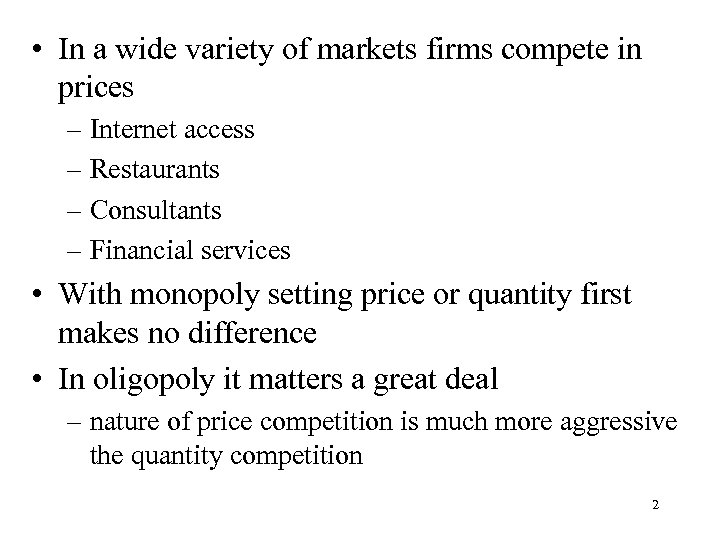• In a wide variety of markets firms compete in prices – Internet access – Restaurants – Consultants – Financial services • With monopoly setting price or quantity first makes no difference • In oligopoly it matters a great deal – nature of price competition is much more aggressive the quantity competition 2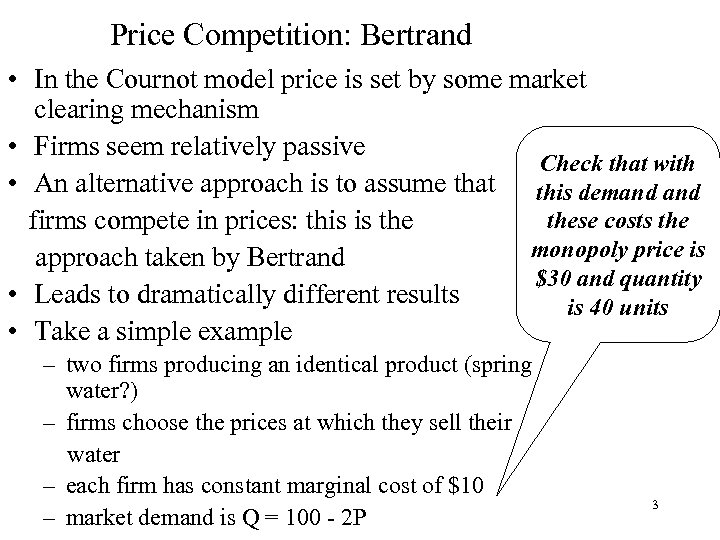Price Competition: Bertrand • In the Cournot model price is set by some market clearing mechanism • Firms seem relatively passive Check that with • An alternative approach is to assume that this demand these costs the firms compete in prices: this is the monopoly price is approach taken by Bertrand \$30 and quantity • Leads to dramatically different results is 40 units • Take a simple example – two firms producing an identical product (spring water? ) – firms choose the prices at which they sell their water – each firm has constant marginal cost of \$10 – market demand is Q = 100 - 2 P 3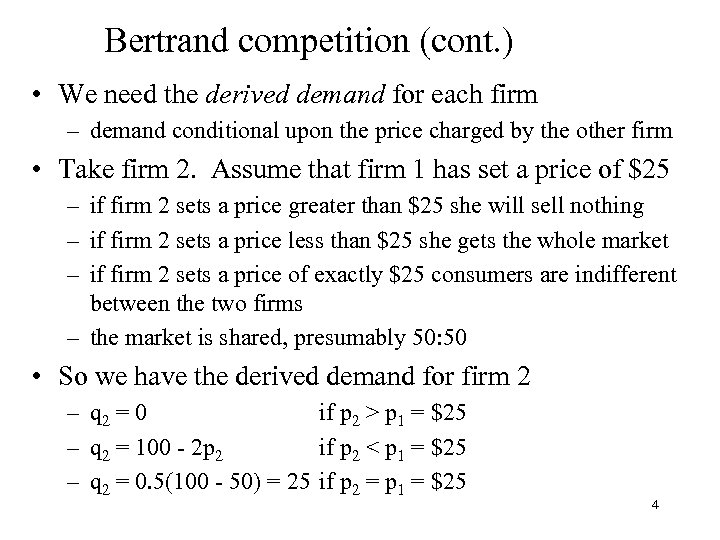Bertrand competition (cont. ) • We need the derived demand for each firm – demand conditional upon the price charged by the other firm • Take firm 2. Assume that firm 1 has set a price of \$25 – if firm 2 sets a price greater than \$25 she will sell nothing – if firm 2 sets a price less than \$25 she gets the whole market – if firm 2 sets a price of exactly \$25 consumers are indifferent between the two firms – the market is shared, presumably 50: 50 • So we have the derived demand for firm 2 – q 2 = 0 if p 2 > p 1 = \$25 – q 2 = 100 - 2 p 2 if p 2 < p 1 = \$25 – q 2 = 0. 5(100 - 50) = 25 if p 2 = p 1 = \$25 4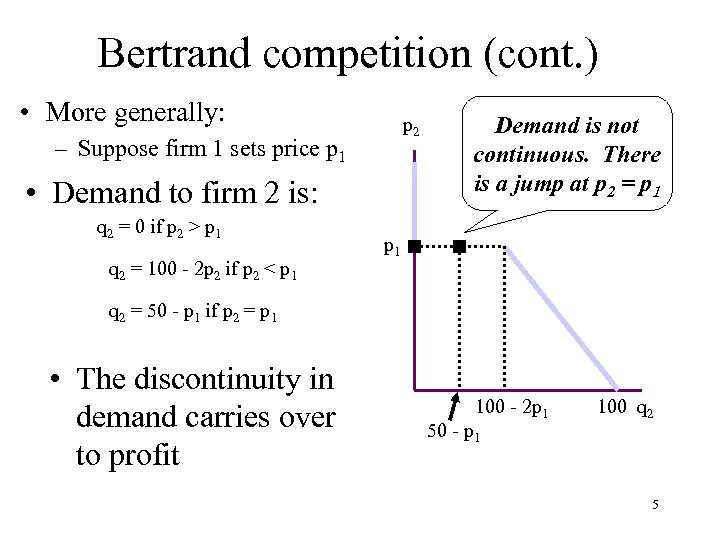Bertrand competition (cont. ) • More generally: p 2 – Suppose firm 1 sets price p 1 • Demand to firm 2 is: q 2 = 0 if p 2 > p 1 q 2 = 100 - 2 p 2 if p 2 < p 1 Demand is not continuous. There is a jump at p 2 = p 1 q 2 = 50 - p 1 if p 2 = p 1 • The discontinuity in demand carries over to profit 100 - 2 p 1 50 - p 1 100 q 2 5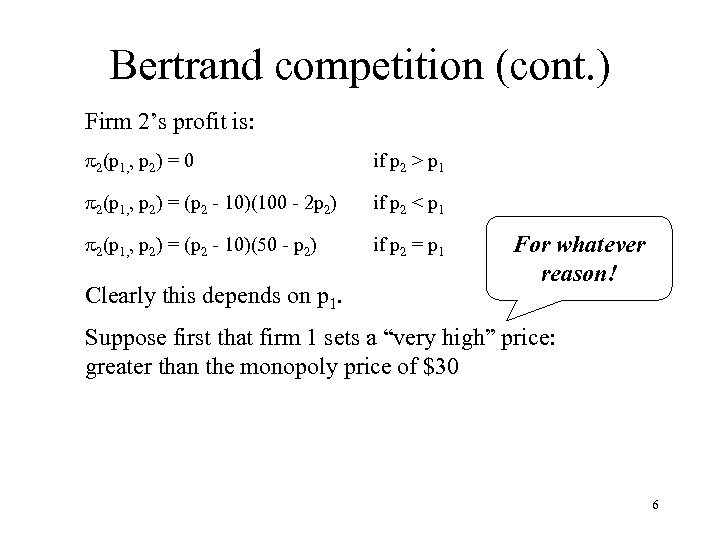Bertrand competition (cont. ) Firm 2’s profit is: p 2(p 1, , p 2) = 0 if p 2 > p 1 p 2(p 1, , p 2) = (p 2 - 10)(100 - 2 p 2) if p 2 < p 1 p 2(p 1, , p 2) = (p 2 - 10)(50 - p 2) if p 2 = p 1 Clearly this depends on p 1. For whatever reason! Suppose first that firm 1 sets a “very high” price: greater than the monopoly price of \$30 6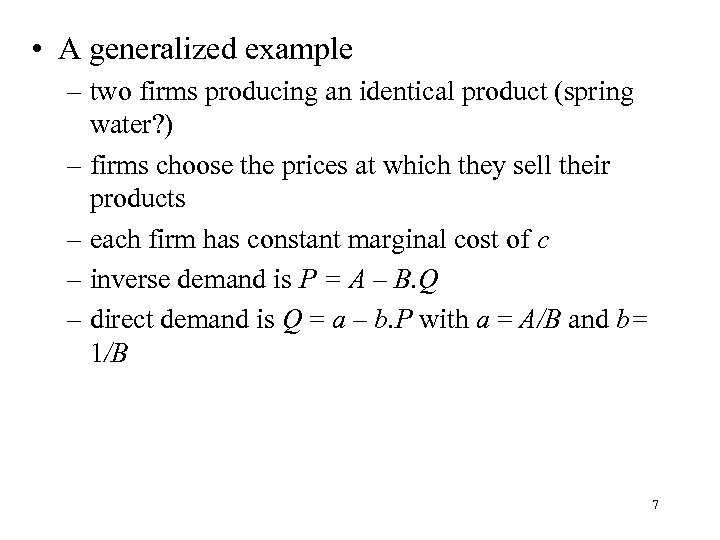• A generalized example – two firms producing an identical product (spring water? ) – firms choose the prices at which they sell their products – each firm has constant marginal cost of c – inverse demand is P = A – B. Q – direct demand is Q = a – b. P with a = A/B and b= 1/B 7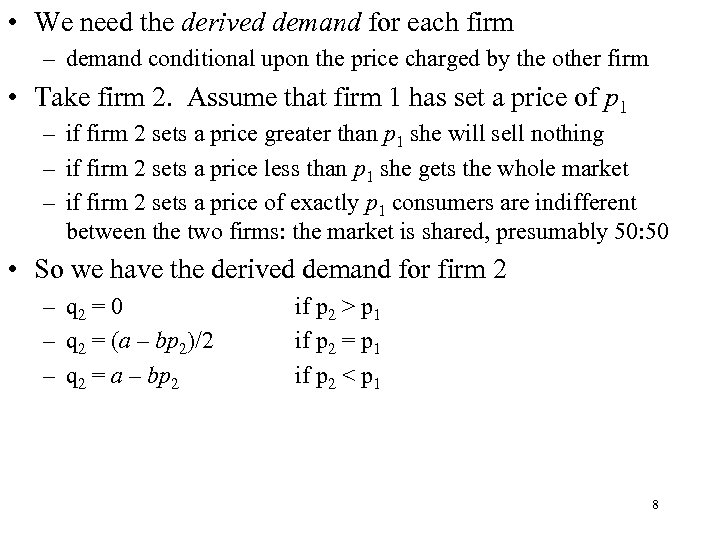• We need the derived demand for each firm – demand conditional upon the price charged by the other firm • Take firm 2. Assume that firm 1 has set a price of p 1 – if firm 2 sets a price greater than p 1 she will sell nothing – if firm 2 sets a price less than p 1 she gets the whole market – if firm 2 sets a price of exactly p 1 consumers are indifferent between the two firms: the market is shared, presumably 50: 50 • So we have the derived demand for firm 2 – q 2 = 0 – q 2 = (a – bp 2)/2 – q 2 = a – bp 2 if p 2 > p 1 if p 2 = p 1 if p 2 < p 1 8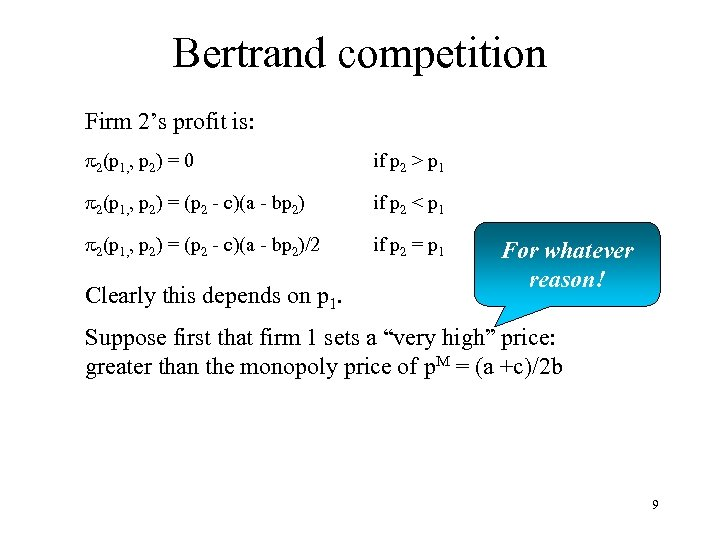Bertrand competition Firm 2’s profit is: p 2(p 1, , p 2) = 0 if p 2 > p 1 p 2(p 1, , p 2) = (p 2 - c)(a - bp 2) if p 2 < p 1 p 2(p 1, , p 2) = (p 2 - c)(a - bp 2)/2 if p 2 = p 1 Clearly this depends on p 1. For whatever reason! Suppose first that firm 1 sets a “very high” price: greater than the monopoly price of p. M = (a +c)/2 b 9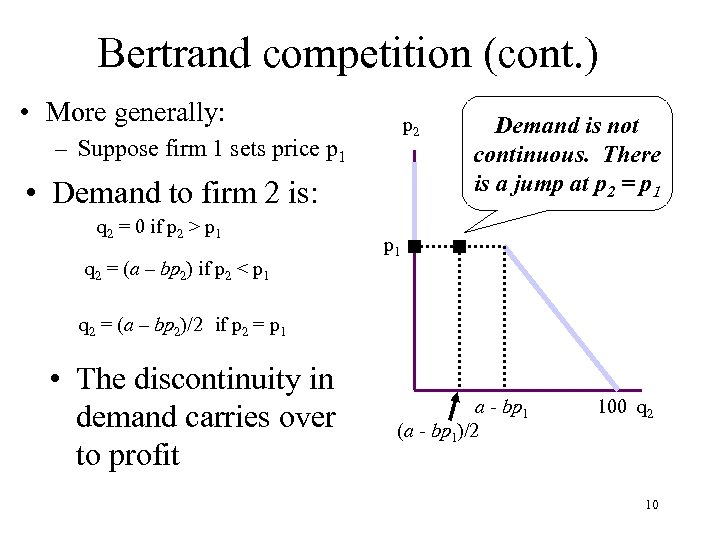Bertrand competition (cont. ) • More generally: p 2 – Suppose firm 1 sets price p 1 • Demand to firm 2 is: q 2 = 0 if p 2 > p 1 q 2 = (a – bp 2) if p 2 < p 1 Demand is not continuous. There is a jump at p 2 = p 1 q 2 = (a – bp 2)/2 if p 2 = p 1 • The discontinuity in demand carries over to profit a - bp 1 (a - bp 1)/2 100 q 2 10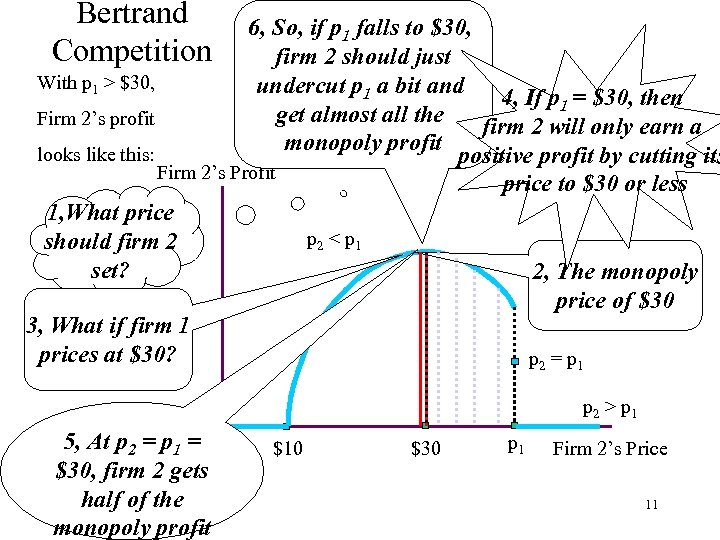Bertrand Competition 6, So, if p 1 falls to \$30, firm 2 should just With p 1 > \$30, undercut p 1 a bit and 4, If p 1 = \$30, then get almost all the Firm 2’s profit firm 2 will only earn a monopoly profit looks like this: positive profit by cutting its Firm 2’s Profit price to \$30 or less 1, What price p 2 < p 1 should firm 2 set? 2, The monopoly price of \$30 3, What if firm 1 prices at \$30? p 2 = p 1 p 2 > p 1 5, At p 2 = p 1 = \$30, firm 2 gets half of the monopoly profit \$10 \$30 p 1 Firm 2’s Price 11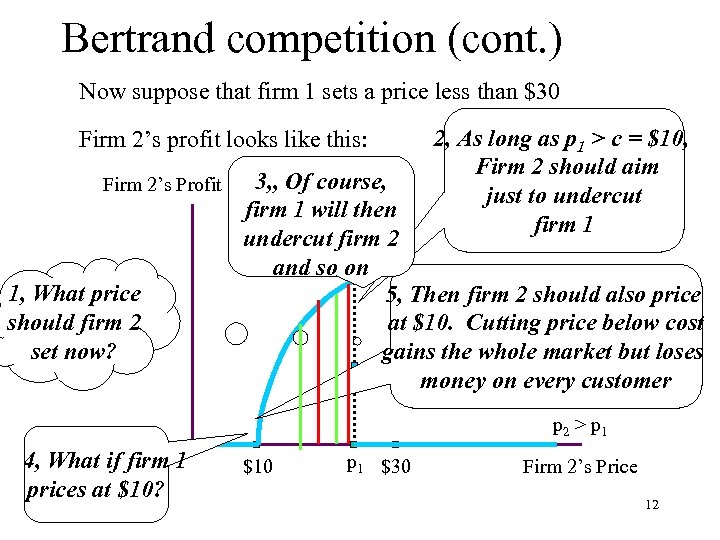Bertrand competition (cont. ) Now suppose that firm 1 sets a price less than \$30 Firm 2’s profit looks like this: Firm 2’s Profit 1, What price should firm 2 set now? 2, As long as p 1 > c = \$10, Firm 2 should aim just to undercut firm 1 3, , Of course, firm 1 will then undercut firm 2 p 2 < p 1 and so on 5, Then firm 2 should also price at \$10. Cutting price below cost gains the whole market but loses p 2 = p 1 money on every customer p 2 > p 1 4, What if firm 1 prices at \$10? \$10 p 1 \$30 Firm 2’s Price 12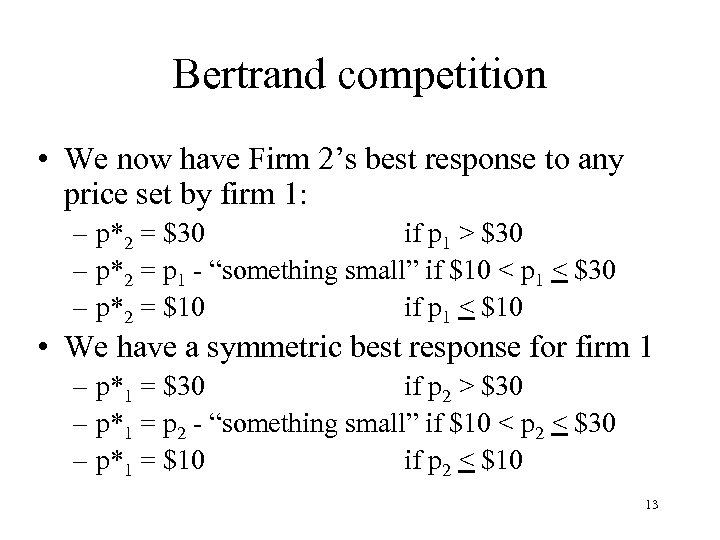Bertrand competition • We now have Firm 2’s best response to any price set by firm 1: – p*2 = \$30 if p 1 > \$30 – p*2 = p 1 - “something small” if \$10 < p 1 < \$30 – p*2 = \$10 if p 1 < \$10 • We have a symmetric best response for firm 1 – p*1 = \$30 if p 2 > \$30 – p*1 = p 2 - “something small” if \$10 < p 2 < \$30 – p*1 = \$10 if p 2 < \$10 13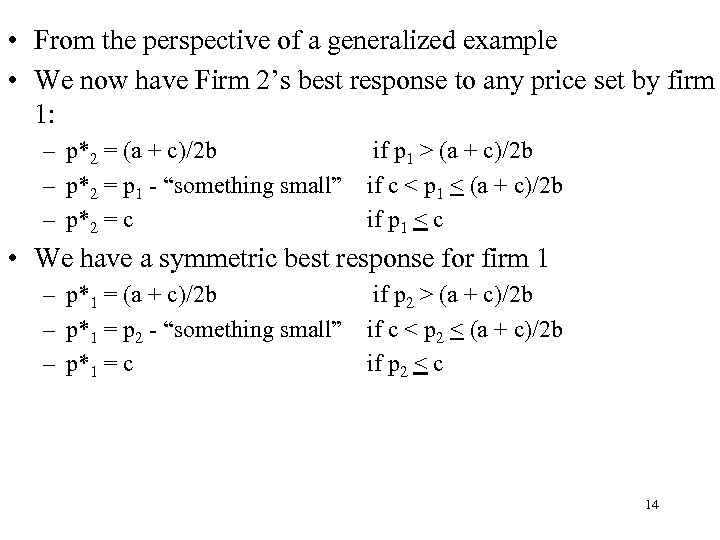• From the perspective of a generalized example • We now have Firm 2’s best response to any price set by firm 1: – p*2 = (a + c)/2 b – p*2 = p 1 - “something small” – p*2 = c if p 1 > (a + c)/2 b if c < p 1 < (a + c)/2 b if p 1 < c • We have a symmetric best response for firm 1 – p*1 = (a + c)/2 b – p*1 = p 2 - “something small” – p*1 = c if p 2 > (a + c)/2 b if c < p 2 < (a + c)/2 b if p 2 < c 14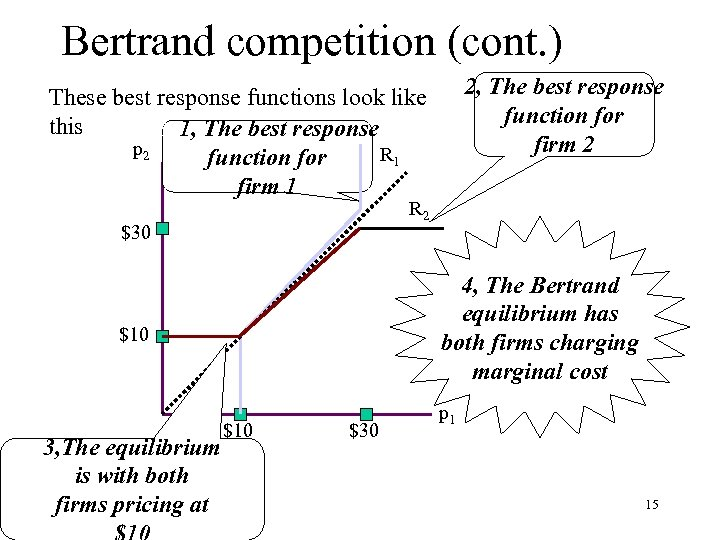Bertrand competition (cont. ) 2, The best response function for firm 2 These best response functions look like this 1, 1, The best response p 2 R 1 function for firm 1 R 2 \$30 4, The Bertrand equilibrium has both firms charging marginal cost \$10 3, The equilibrium is with both firms pricing at \$10 \$30 p 1 15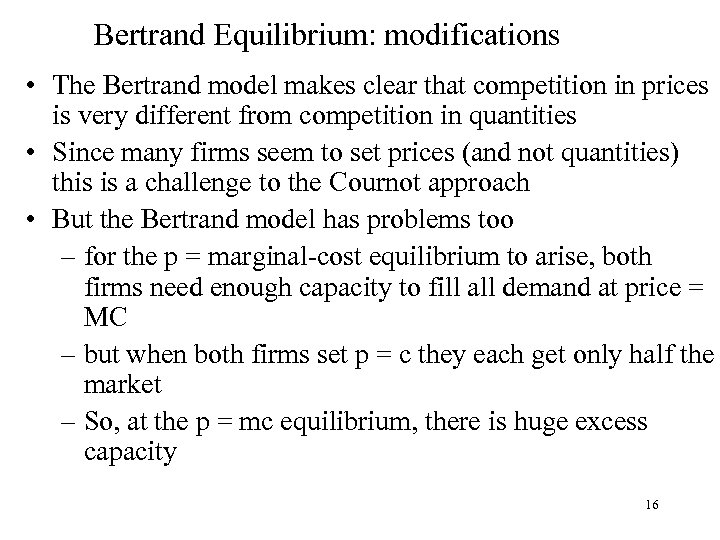Bertrand Equilibrium: modifications • The Bertrand model makes clear that competition in prices is very different from competition in quantities • Since many firms seem to set prices (and not quantities) this is a challenge to the Cournot approach • But the Bertrand model has problems too – for the p = marginal-cost equilibrium to arise, both firms need enough capacity to fill all demand at price = MC – but when both firms set p = c they each get only half the market – So, at the p = mc equilibrium, there is huge excess capacity 16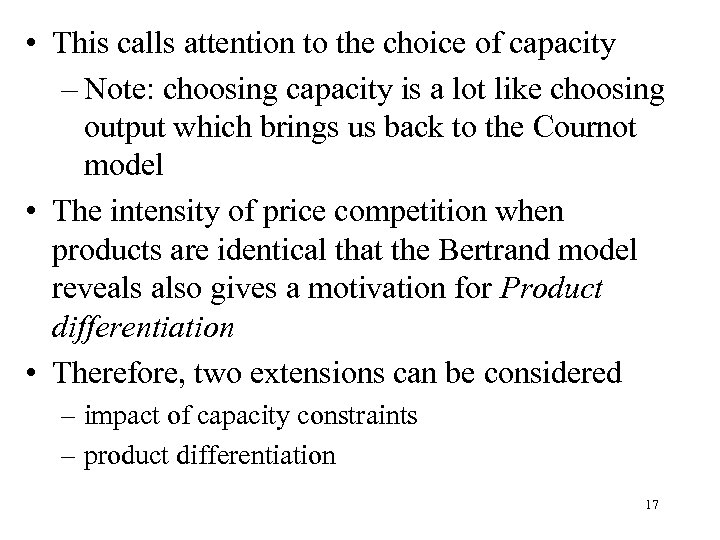• This calls attention to the choice of capacity – Note: choosing capacity is a lot like choosing output which brings us back to the Cournot model • The intensity of price competition when products are identical that the Bertrand model reveals also gives a motivation for Product differentiation • Therefore, two extensions can be considered – impact of capacity constraints – product differentiation 17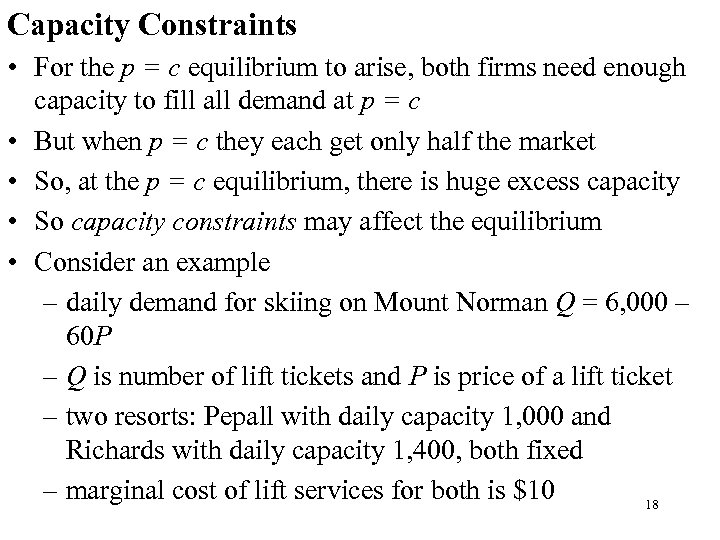Capacity Constraints • For the p = c equilibrium to arise, both firms need enough capacity to fill all demand at p = c • But when p = c they each get only half the market • So, at the p = c equilibrium, there is huge excess capacity • So capacity constraints may affect the equilibrium • Consider an example – daily demand for skiing on Mount Norman Q = 6, 000 – 60 P – Q is number of lift tickets and P is price of a lift ticket – two resorts: Pepall with daily capacity 1, 000 and Richards with daily capacity 1, 400, both fixed – marginal cost of lift services for both is \$10 18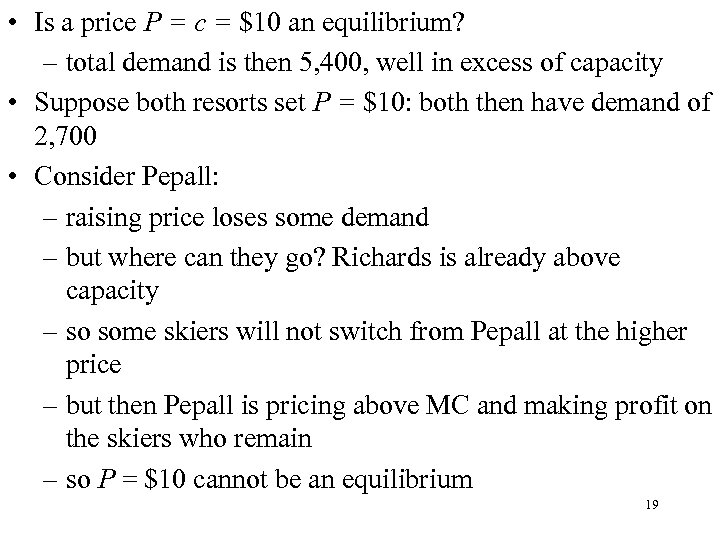• Is a price P = c = \$10 an equilibrium? – total demand is then 5, 400, well in excess of capacity • Suppose both resorts set P = \$10: both then have demand of 2, 700 • Consider Pepall: – raising price loses some demand – but where can they go? Richards is already above capacity – so some skiers will not switch from Pepall at the higher price – but then Pepall is pricing above MC and making profit on the skiers who remain – so P = \$10 cannot be an equilibrium 19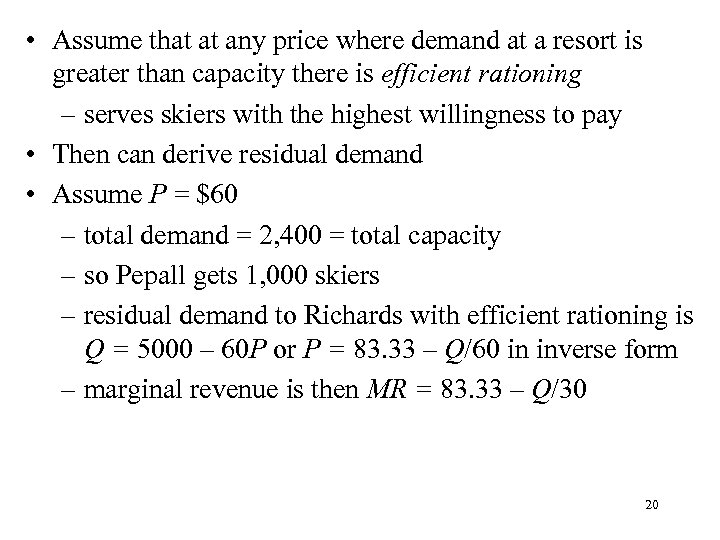• Assume that at any price where demand at a resort is greater than capacity there is efficient rationing – serves skiers with the highest willingness to pay • Then can derive residual demand • Assume P = \$60 – total demand = 2, 400 = total capacity – so Pepall gets 1, 000 skiers – residual demand to Richards with efficient rationing is Q = 5000 – 60 P or P = 83. 33 – Q/60 in inverse form – marginal revenue is then MR = 83. 33 – Q/30 20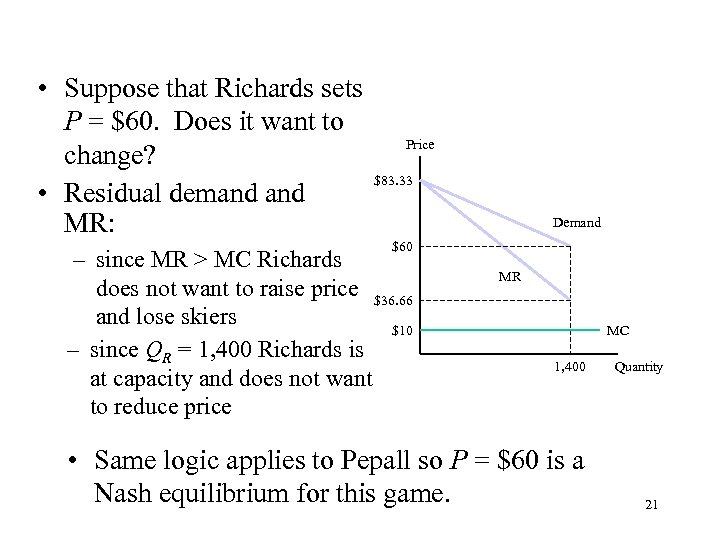• Suppose that Richards sets P = \$60. Does it want to change? • Residual demand MR: Price \$83. 33 Demand \$60 – since MR > MC Richards does not want to raise price \$36. 66 and lose skiers \$10 – since QR = 1, 400 Richards is at capacity and does not want to reduce price MR MC 1, 400 • Same logic applies to Pepall so P = \$60 is a Nash equilibrium for this game. Quantity 21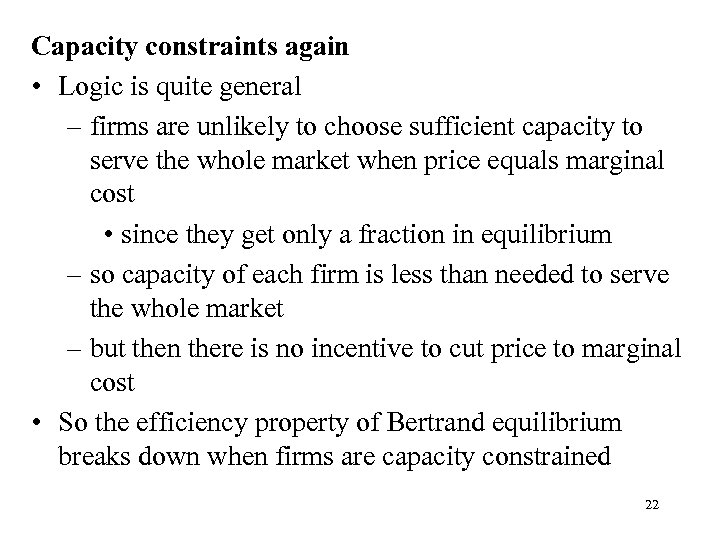Capacity constraints again • Logic is quite general – firms are unlikely to choose sufficient capacity to serve the whole market when price equals marginal cost • since they get only a fraction in equilibrium – so capacity of each firm is less than needed to serve the whole market – but then there is no incentive to cut price to marginal cost • So the efficiency property of Bertrand equilibrium breaks down when firms are capacity constrained 22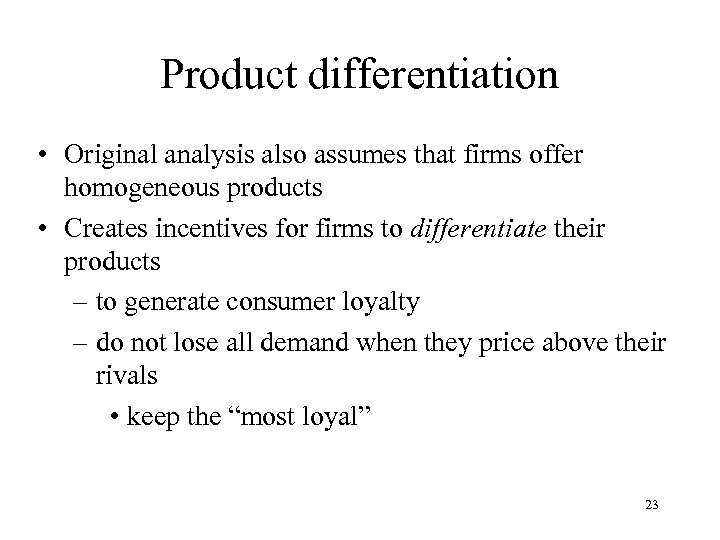Product differentiation • Original analysis also assumes that firms offer homogeneous products • Creates incentives for firms to differentiate their products – to generate consumer loyalty – do not lose all demand when they price above their rivals • keep the “most loyal” 23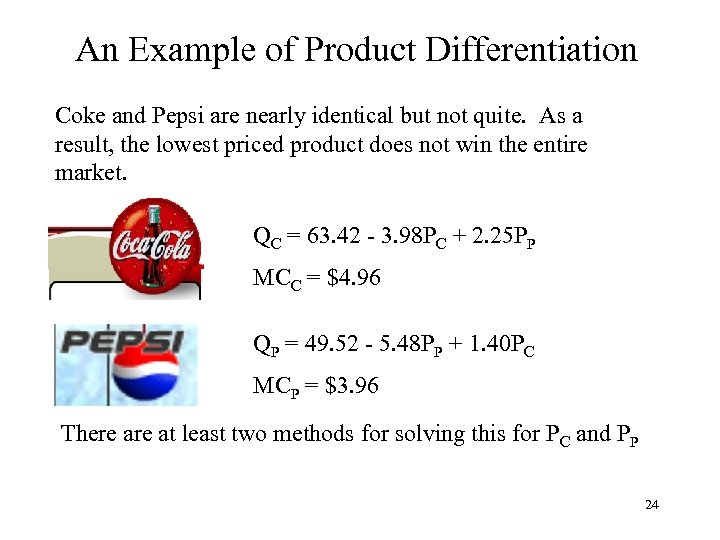An Example of Product Differentiation Coke and Pepsi are nearly identical but not quite. As a result, the lowest priced product does not win the entire market. QC = 63. 42 - 3. 98 PC + 2. 25 PP MCC = \$4. 96 QP = 49. 52 - 5. 48 PP + 1. 40 PC MCP = \$3. 96 There at least two methods for solving this for PC and PP 24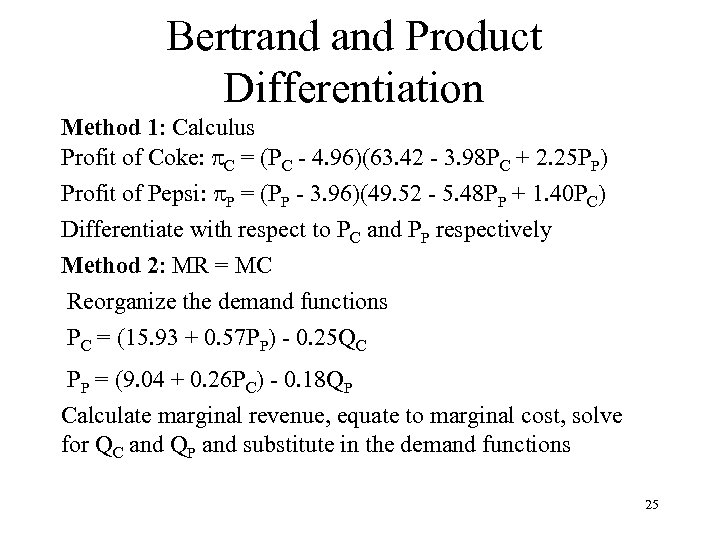Bertrand Product Differentiation Method 1: Calculus Profit of Coke: p. C = (PC - 4. 96)(63. 42 - 3. 98 PC + 2. 25 PP) Profit of Pepsi: p. P = (PP - 3. 96)(49. 52 - 5. 48 PP + 1. 40 PC) Differentiate with respect to PC and PP respectively Method 2: MR = MC Reorganize the demand functions PC = (15. 93 + 0. 57 PP) - 0. 25 QC PP = (9. 04 + 0. 26 PC) - 0. 18 QP Calculate marginal revenue, equate to marginal cost, solve for QC and QP and substitute in the demand functions 25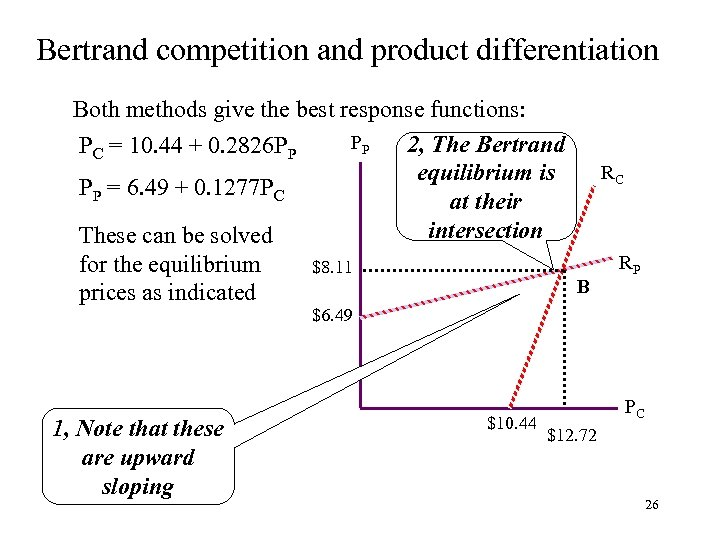Bertrand competition and product differentiation Both methods give the best response functions: PP 2, The Bertrand PC = 10. 44 + 0. 2826 PP RC equilibrium is PP = 6. 49 + 0. 1277 PC at their intersection These can be solved RP for the equilibrium \$8. 11 B prices as indicated \$6. 49 1, Note that these are upward sloping \$10. 44 PC \$12. 72 26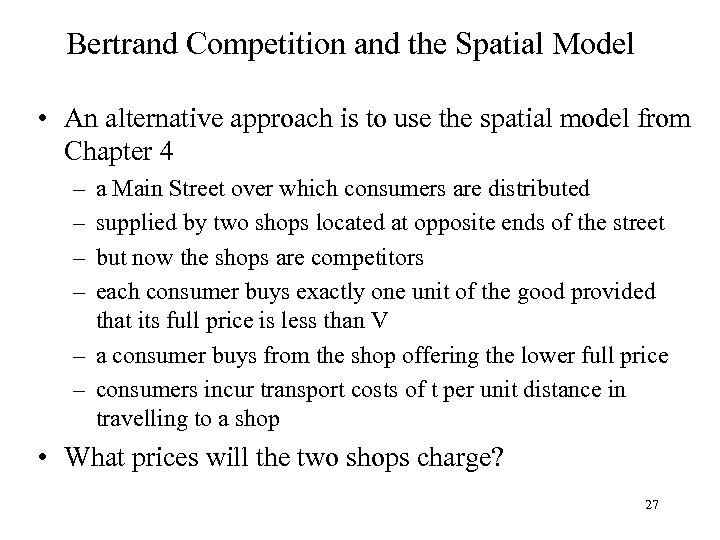Bertrand Competition and the Spatial Model • An alternative approach is to use the spatial model from Chapter 4 – – a Main Street over which consumers are distributed supplied by two shops located at opposite ends of the street but now the shops are competitors each consumer buys exactly one unit of the good provided that its full price is less than V – a consumer buys from the shop offering the lower full price – consumers incur transport costs of t per unit distance in travelling to a shop • What prices will the two shops charge? 27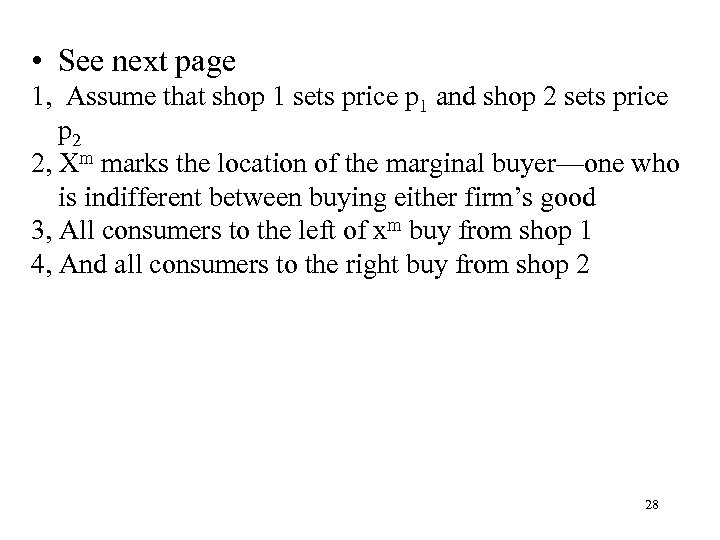• See next page 1, Assume that shop 1 sets price p 1 and shop 2 sets price p 2 2, Xm marks the location of the marginal buyer—one who is indifferent between buying either firm’s good 3, All consumers to the left of xm buy from shop 1 4, And all consumers to the right buy from shop 2 28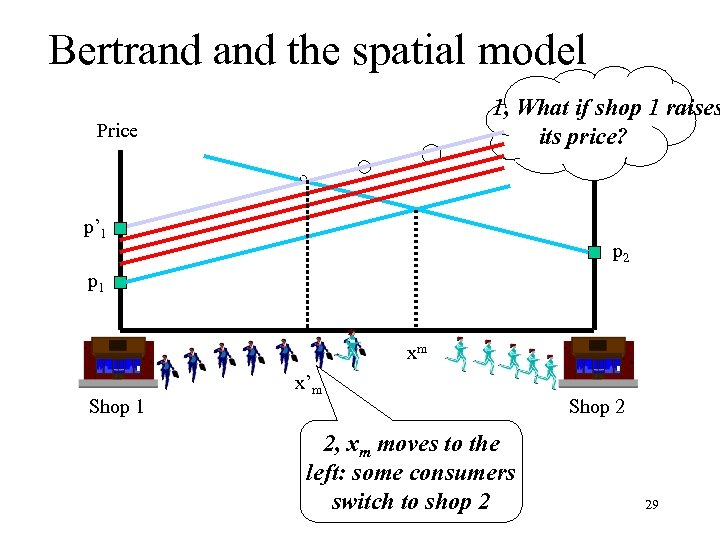Bertrand the spatial model 1, What if shop 1 raises Price its price? Price p’ 1 p 2 p 1 xm Shop 1 x’m 2, xm moves to the left: some consumers switch to shop 2 Shop 2 29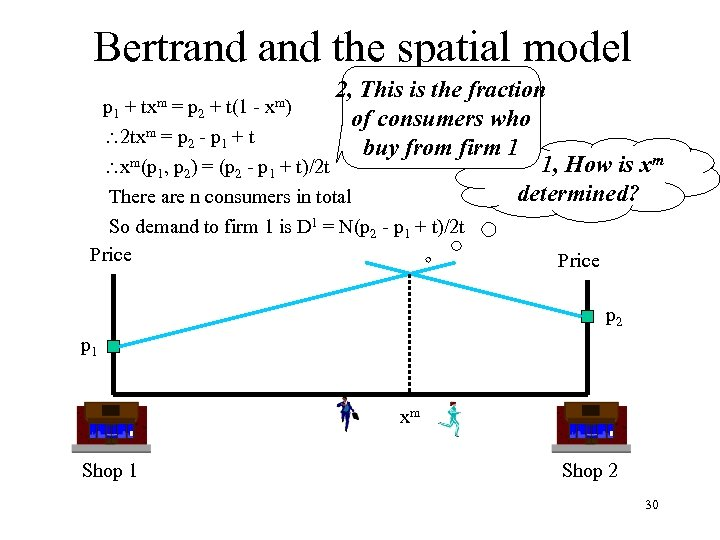Bertrand the spatial model 2, This is the fraction p 1 + = p 2 + t(1 of consumers who m=p -p +t 2 tx 2 1 buy from firm 1 m(p , p ) = (p - p + t)/2 t 1, How is xm x 1 2 2 1 determined? There are n consumers in total txm xm) So demand to firm 1 is D 1 = N(p 2 - p 1 + t)/2 t Price p 2 p 1 xm Shop 1 Shop 2 30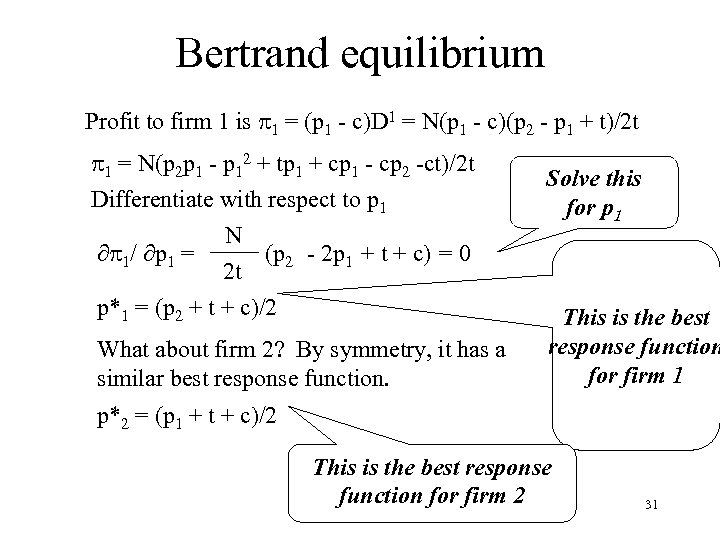Bertrand equilibrium Profit to firm 1 is p 1 = (p 1 - c)D 1 = N(p 1 - c)(p 2 - p 1 + t)/2 t p 1 = N(p 2 p 1 - p 12 + tp 1 + cp 1 - cp 2 -ct)/2 t Differentiate with respect to p 1 N p 1/ p 1 = (p 2 - 2 p 1 + t + c) = 0 2 t p*1 = (p 2 + t + c)/2 What about firm 2? By symmetry, it has a similar best response function. Solve this for p 1 This is the best response function for firm 1 p*2 = (p 1 + t + c)/2 This is the best response function for firm 2 31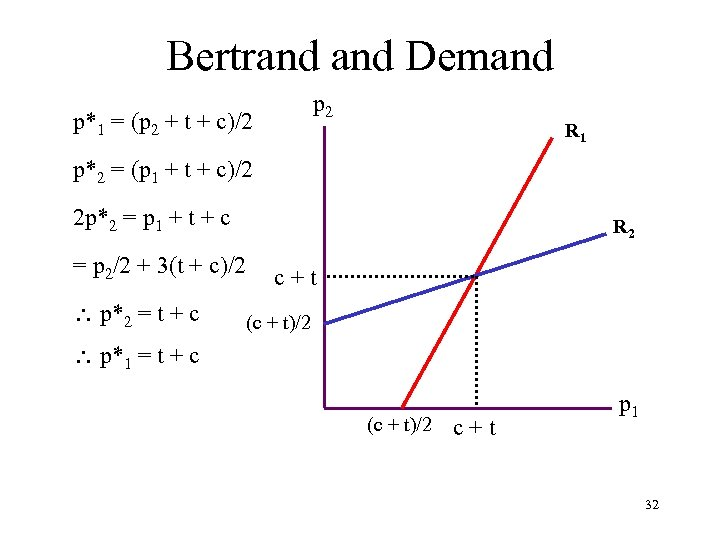Bertrand Demand p 2 p*1 = (p 2 + t + c)/2 R 1 p*2 = (p 1 + t + c)/2 2 p*2 = p 1 + t + c R 2 = p 2/2 + 3(t + c)/2 p*2 = t + c c+t (c + t)/2 p*1 = t + c (c + t)/2 c+t p 1 3233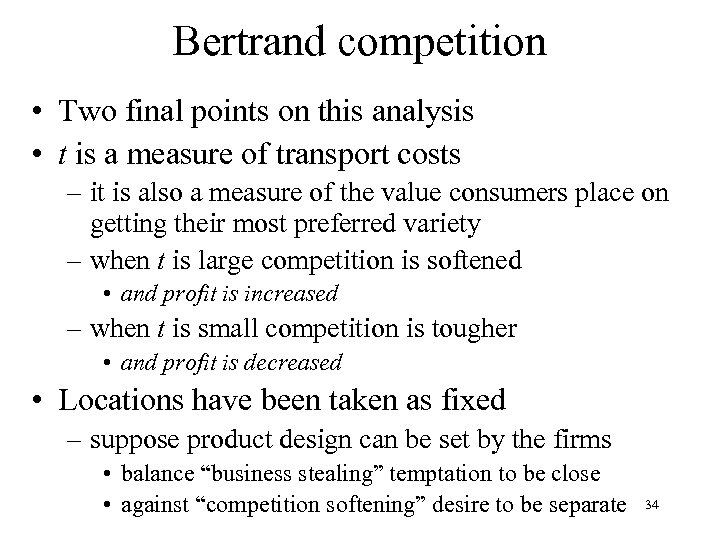Bertrand competition • Two final points on this analysis • t is a measure of transport costs – it is also a measure of the value consumers place on getting their most preferred variety – when t is large competition is softened • and profit is increased – when t is small competition is tougher • and profit is decreased • Locations have been taken as fixed – suppose product design can be set by the firms • balance “business stealing” temptation to be close • against “competition softening” desire to be separate 34